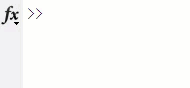In Matlab programming, the for loop is a frequently used statement for iterating on arrays. When the array is very large, the execution time of for-loop may be considerably long, and we often expect to obtain information about the executed proportion. Based on this, I wrote the following code to achieve this function.

Contents

## Sample output## The snippet of code

N = 10000;

percents = '';
for i=1:N
if (mod(i-[1,0],(N*0.01))*[1;-1]) > 0
fprintf(repmat('\b', 1, numel(percents)));
percents = sprintf('Percentage: %.0f%%',i/N*100);
fprintf('%s',percents);
end
% other user codes ...
pause(0.001)
% other user codes ...
end
fprintf('\n');

## Explanation

The above code contains the basic realization ideas, you may need to modify these codes to apply them to your project.

The following code is used to detect the jump of the one-hundredth of the array size interval, and the if condition is met every time the count value i exceeds the one-hundredth intervals.

if (mod(i-[1,0],(N*0.01))*[1;-1]) > 0

Then, the'\b' character is used to clear the last fprintf output to maintain the display position of percentage information.

Categories: Matlab